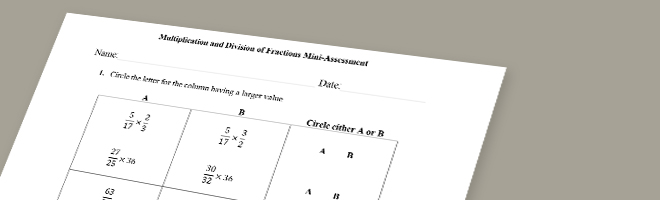• 01/08/15   |   Adjusted: 10/09/19   |   2 files# Multiplication and Division of Fractions Mini-Assessment

Author: Student Achievement Partners

• Description
• Files

This mini-assessment is designed to illustrate cluster 5.NF.B, which sets an expectation for students to apply and extend previous understandings of multiplication and division to multiply and divide fractions. This mini-assessment is designed for teachers to use either in the classroom, for self-learning, or in professional development settings to:

• Evaluate students' understanding of 5.NF.B in order to prepare to teach this material or to check for student ability to demonstrate understanding and apply these concepts;
• Gain knowledge about assessing conceptual understanding of fraction multiplication and division; and
• Illustrate CCSS-aligned assessment problems.

• Making the Shifts

How does this mini-assessment exemplify the instructional Shifts required by CCSSM?

 Focus Belongs to the major work of fifth grade Coherence Extends grade 4 understanding of multiplication of fractions by whole numbers to grade 5 understanding of multiplication of fractions or whole numbers by fractions Rigor Conceptual Understanding: primary in this mini-assessment Procedural Skill and Fluency: not targeted in this mini-assessment Application: not targeted in this mini-assessment
• Noteworthy features of this resource
• Mathematically:
• Addresses the conceptual understanding described in 5.NF.B mostly using short questions that are either free of context or have little context
• Connects the concept of fractions to division
• Extends understanding of division to divide unit fractions by whole numbers and whole numbers by unit fractions
• As a mini-assessment:
• Assesses important concepts directly, like knowing that any fraction multiplied by a number greater than 1 will result in a product that is larger than the given fraction
• Uses a mix of short conceptual problems with problems that require longer chains of reasoning
• Offers item-level annotations for some of the problems describing how they assess conceptual understanding